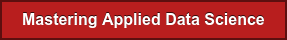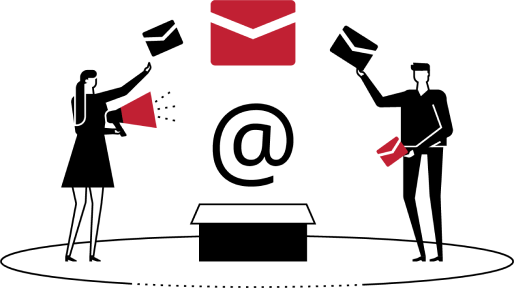# What is machine learning?

You want to learn or know more about machine learning? Let's talk about unsupervised, supervised and reinforment earning to make a prediction.Machine learning is a subfield of computer science that evolved from the study of pattern recognition and computational theory in artificial intelligence and data science. In 1959, Arthur Samuel defined it as a "Field of study that gives computers the ability to learn without being explicitly programmed".

Machine learning explores the study and construction of algorithms that can learn from and make predictions on data. Such algorithms operate by building a model from example inputs in order to make data-driven predictions or decisions, rather than following strictly static program instructions.The three different learning types to make prediction about the future outcome:

## 1. Supervised

The main purpose is to learn the model from labeled training data that allow us to make predictions. The predictions are about unseen or future projections.

The term supervised learning refer to set of samples where the desired output labels are already known. For example, consider email spam filtering, we can train a model using a supervised machine learning algorithm.

The machine learning will detect the label of email, the email which is correctly marked as spam or not spam.

To predict whether a new email belongs to either category. A supervised learning task with discrete class labels, such as in the previous e-mail filter example it is called classification task.

Another subcategory of supervised learning is regression, where the outcome signal is a continuous value.

Classification for predicting class labels

Classification model is known as a subcategory of supervised learning. The goal is to predict the categorical class labels of new instances of class labels based on past observations. The class labels here are discrete, the unordered values that can be understood as the group membership’s format of the instances. The e-mail spam detection represents a typical example of a binary classification task, where the machine learning algorithm learns a set of rules in order to distinguish between two possible classes: spam and non-spam email.

However, the set of class labels does not have to be of a binary nature. The predictive model learned by an algorithm can assign any class label that was presented in the training dataset to a new, unlabeled instance. A typical example of a multi-class classification task is handwritten character recognition.

Supervised Learning Regression for predicting continuous outcomes. In the previous section that the task of classification is to assign categorical, unordered labels to instances. The second type of supervised learning is the prediction of continuous outcomes, which is also called regression analysis. In regression analysis, we are given a number of the predictor (explanatory) variables and a continuous response variable (outcome), and we try to find a relationship between those variables that allows us to predict an outcome.

## 2. Unsupervised

Discovering hidden structures with unsupervised learning in supervised learning, we know the right answer beforehand when we train our model, and in reinforcement learning, we define a measure of reward for particular actions by the agent. In unsupervised learning, however, we are dealing with unlabeled data or data of the unknown structure. Using unsupervised learning techniques, we are able to explore the structure of our data to extract meaningful information without the guidance of a known outcome variable or reward function. Finding subgroups with clustering Clustering is an exploratory data analysis technique that allows us to organize a pile of information into meaningful subgroups (clusters) without having any prior knowledge of their group memberships. Each cluster that may arise during the analysis defines a group of objects that share a certain degree of similarity but are more dissimilar to objects in other clusters, which is why clustering is also sometimes called "unsupervised classification." Clustering is a great technique for structuring information and deriving meaningful relationships among data, For example, it allows marketers to discover customer groups based on their interests in order to develop distinct marketing programs.

## 3. Reinforcement

We develop a system (agent) that improve the performance of algorithm based on interaction with another variable in the environment, this is machine learning. The information about the current environment is typical included so the reward signal. We can consider reinforcement of current environment and agent rebuilding the algorithm as supervised learning.

However feedback is not 100% accurate at data environment labels. It can be considered as a good measure of how well the reinforcement learning was applied. We can calculate the reward function. In this case, an agent can then use reinforcement learning to learn a series of actions that maximizes this accuracy by exploratory trial-and-error approach or deliberative planning. One of the most popular examples of reinforcement learning is a chess engine. In this case, the agent decides upon a series of moves depending on the state of the board (the environment), and the reward can be defined as win or lose at the end.## Have any questions?